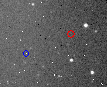# Brightness Analysis Nov 11, 2002 ImagesFor the Red light persona only, and based on percentage of brightness to background for each date. Difficulty finding candidates in Sept 21 2002 and Oct 11 2002 because of flaring in most candidates, which skews the strength test results for those dates. White has proved a better indicator of increased brightness. Details in tables below.

Summary:

`Oct  4 2002 = 143% `

Summary of Frames for Nov 11 2002

```Frame 1	     = 148 %
Frame 11 (a) = 147 %
Frame 11 (b) = 166 %
Frame 14     = 134 %
Frame 15     = 149 %
Frame 18     = 191 %
Frame 20     = 150 %
Total Combined ---
and Averaged   155 % ```

It does indicate increasing brightness.

Regards, J. William Dell

Oct 4 2002 – Frame 4

 Red candidate just under very bright Star ( Possible Microlensing event) 114 104 117 109 120 153 132 113 113 236 141 126 110 121 113 109 Total Surrounding 1392 / 12 = 116 153/ 116 = 131.8966 236/ 116 = 203.4483 132/ 116 = 113.7931 141/ 166 = 121.5517 Average % 142.6724

Nov 11 2002 Frames

 Frame 1 Red 3530 3571 3493 3456 3682 5443 4070 3737 3516 7388 4179 3599 3619 3583 3537 3497 Total Surround 42820 / 12 = 3568.333 5443/ 3568 = 152.5504 7388/ 3568 = 207.0628 4070/ 3568 = 114.0695 4179/ 3568 = 117.1244 Average % 147.7018

 Frame 11 Red (offset to Frame 1 red spot) 3514 3477 3672 3810 3754 4247 7662 3504 3832 3947 4352 3653 3690 3570 3683 Total Surround 44106 / 12 = 3675.5 7662/ 3675 = 208.4898 4247/ 3675 = 115.5646 4352/ 3675 = 118.4218 Average % 147.4921

Frame 11 Red (2nd Candidate, Just below first)

 3607 3565 3546 3632 3514 4366 7585 3629 3780 3639 3603 3396 Total Surround 35911 / 10 = 3591.1 4366/ 3591 = 121.5817 7585/ 3591 = 211.2225 Average % 166.4021 Frame 14 Red (Inside Triangle) 2786 3232 3083 3324 3190 4095 4744 3204 3360 4070 3269 3259 3190 3219 3307 Total Surrounding 38423 / 12 3201.917 4095/ 3201 = 127.9288 4070/ 3201 = 127.1478 4744/ 3201 148.2037 Average % 134.4267 Frame 15 Red (Inside Triangle Avis Recognizzed) 1721 1707 1731 1670 1696 3381 2221 1684 1707 2454 2170 1675 1808 1763 1701 1761 Total Surrounding 20624 / 12 = 1718.667 3381/ 1718 = 196.7986 2454/ 1718 = 142.8405 2221/ 1718 = 129.2782 2170/ 1718 = 126.3097 Average % 148.806 Frame 18 Red 1739 1628 1740 1661 1732 3395 3923 1633 1650 1792 2346 1658 1649 1707 1689 Total Surrounding 20278 / 12 = 1689.833 3395/ 1689 = 201.0065 3923 / 1689 = 232.2676 2346/ 1689 = 138.8988 Average % 190.7243

Frame 20 Red (Above Triangle 3 Pixel, 3rd pixel close to Background)

 1837 1859 1883 1851 2656 1825 1801 1821 3685 1945 1869 1871 1770 1837 1909 Total Surrounding 22133 / 12 = 1844.417 2656 / 1844 = 144.0347 3685/ 1844 = 199.8373 1945 / 1844 = 105.4772 Average % 149.7831